## BlogPerformance Metrics for Classification problems in Machine Learning

After doing the usual Feature Engineering, Selection, and of course, implementing a model and getting some output in forms of a probability or a class, the next step is to find out how effective is the model based on some metric using test datasets. Different performance metrics are used to evaluate different Machine Learning Algorithms. For now, we will be focusing on the ones used for Classification problems. We can use classification performance metrics such as Log-Loss, Accuracy, AUC(Area under Curve) etc. Another example of metric for evaluation of machine learning algorithms is precision, recall, which can be used for sorting algorithms primarily used by search engines.

The metrics that you choose to evaluate your machine learning model is very important. Choice of metrics influences how the performance of machine learning algorithms is measured and compared. Before wasting any more time, let’s jump right in and see what those metrics are.

### 1. Confusion Matrix:

The Confusion matrix is one of the most intuitive and easiest (unless of course, you are not confused)metrics used for finding the correctness and accuracy of the model. It is used for Classification problem where the output can be of two or more types of classes.

Before diving into what the confusion matrix is all about and what it conveys, Let’s say we are solving a classification problem where we are predicting whether a person is having cancer or not.

Let’s give a label of to our target variable:
1: When a person is having cancer 0: When a person is NOT having cancer.

Alright! Now that we have identified the problem, the confusion matrix, is a table with two dimensions (“Actual” and “Predicted”), and sets of “classes” in both dimensions. Our Actual classifications are columns and Predicted ones are Rows.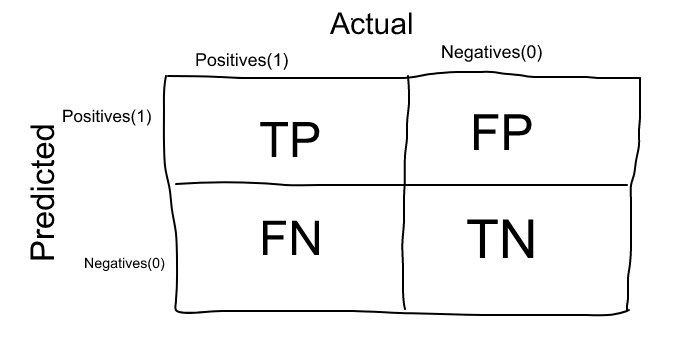Confusion Matrix

The Confusion matrix in itself is not a performance measure as such, but almost all of the performance metrics are based on Confusion Matrix and the numbers inside it.

### Terms associated with Confusion matrix:

1. True Positives (TP): True positives are the cases when the actual class of the data point was 1(True) and the predicted is also 1(True)

Ex: The case where a person is actually having cancer(1) and the model classifying his case as cancer(1) comes under True positive.

2. True Negatives (TN): True negatives are the cases when the actual class of the data point was 0(False) and the predicted is also 0(False

Ex: The case where a person NOT having cancer and the model classifying his case as Not cancer comes under True Negatives.

3. False Positives (FP): False positives are the cases when the actual class of the data point was 0(False) and the predicted is 1(True). False is because the model has predicted incorrectly and positive because the class predicted was a positive one. (1)

Ex: A person NOT having cancer and the model classifying his case as cancer comes under False Positives.

4. False Negatives (FN): False negatives are the cases when the actual class of the data point was 1(True) and the predicted is 0(False). False is because the model has predicted incorrectly and negative because the class predicted was a negative one. (0)

Ex: A person having cancer and the model classifying his case as No-cancer comes under False Negatives.

The ideal scenario that we all want is that the model should give 0 False Positives and 0 False Negatives. But that’s not the case in real life as any model will NOT be 100% accurate most of the times.

When to minimise what?

We know that there will be some error associated with every model that we use for predicting the true class of the target variable. This will result in False Positives and False Negatives(i.e Model classifying things incorrectly as compared to the actual class).

There’s no hard rule that says what should be minimised in all the situations. It purely depends on the business needs and the context of the problem you are trying to solve. Based on that, we might want to minimise either False Positives or False negatives.

1. Minimising False Negatives:

Let’s say in our cancer detection problem example, out of 100 people, only 5 people have cancer. In this case, we want to correctly classify all the cancerous patients as even a very BAD model(Predicting everyone as NON-Cancerous) will give us a 95% accuracy(will come to what accuracy is). But, in order to capture all cancer cases, we might end up making a classification when the person actually NOT having cancer is classified as Cancerous. This might be okay as it is less dangerous than NOT identifying/capturing a cancerous patient since we will anyway send the cancer cases for further examination and reports. But missing a cancer patient will be a huge mistake as no further examination will be done on them.

2. Minimising False Positives:

For better understanding of False Positives, let’s use a different example where the model classifies whether an email is spam or not

Let’s say that you are expecting an important email like hearing back from a recruiter or awaiting an admit letter from a university. Let’s assign a label to the target variable and say,1: “Email is a spam” and 0:”Email is not a spam”

Suppose the Model classifies that important email that you are desperately waiting for, as Spam(case of False positive). Now, in this situation, this is pretty bad than classifying a spam email as important or not spam since in that case, we can still go ahead and manually delete it and it’s not a pain if it happens once a while. So in case of Spam email classification, minimising False positives is more important than False Negatives.

### 2. Accuracy:

Accuracy in classification problems is the number of correct predictions made by the model over all kinds predictions made.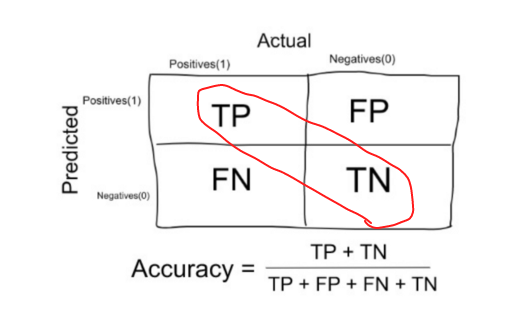Accuracy

In the Numerator, are our correct predictions (True positives and True Negatives)(Marked as red in the fig above) and in the denominator, are the kind of all predictions made by the algorithm(Right as well as wrong ones).

When to use Accuracy:

Accuracy is a good measure when the target variable classes in the data are nearly balanced.

Ex:60% classes in our fruits images data are apple and 40% are oranges.

A model which predicts whether a new image is Apple or an Orange, 97% of times correctly is a very good measure in this example.

When NOT to use Accuracy:

Accuracy should NEVER be used as a measure when the target variable classes in the data are a majority of one class.

Ex: In our cancer detection example with 100 people, only 5 people has cancer. Let’s say our model is very bad and predicts every case as No Cancer. In doing so, it has classified those 95 non-cancer patients correctly and 5 cancerous patients as Non-cancerous. Now even though the model is terrible at predicting cancer, The accuracy of such a bad model is also 95%.

### 3. Precision:

Let’s use the same confusion matrix as the one we used before for our cancer detection example.Precision

Precision is a measure that tells us what proportion of patients that we diagnosed as having cancer, actually had cancer. The predicted positives (People predicted as cancerous are TP and FP) and the people actually having a cancer are TP.

Ex: In our cancer example with 100 people, only 5 people have cancer. Let’s say our model is very bad and predicts every case as Cancer. Since we are predicting everyone as having cancer, our denominator(True positives and False Positives) is 100 and the numerator, person having cancer and the model predicting his case as cancer is 5. So in this example, we can say that Precision of such model is 5%.

### 4. Recall or Sensitivity: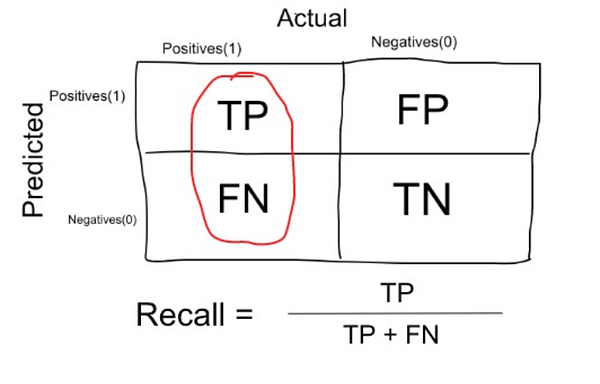Recall or Sensitivity

Recall is a measure that tells us what proportion of patients that actually had cancer was diagnosed by the algorithm as having cancer. The actual positives (People having cancer are TP and FN) and the people diagnosed by the model having a cancer are TP. (Note: FN is included because the Person actually had a cancer even though the model predicted otherwise).

Ex: In our cancer example with 100 people, 5 people actually have cancer. Let’s say that the model predicts every case as cancer.

So our denominator(True positives and False Negatives) is 5 and the numerator, person having cancer and the model predicting his case as cancer is also 5(Since we predicted 5 cancer cases correctly). So in this example, we can say that the Recall of such model is 100%. And Precision of such a model(As we saw above) is 5%

When to use Precision and When to use Recall?:

It is clear that recall gives us information about a classifier’s performance with respect to false negatives (how many did we miss), while precision gives us information about its performance with respect to false positives(how many did we caught).

Precision is about being precise. So even if we managed to capture only one cancer case, and we captured it correctly, then we are 100% precise.

Recall is not so much about capturing cases correctly but more about capturing all cases that have “cancer” with the answer as “cancer”. So if we simply always say every case as “cancer”, we have 100% recall.

So basically if we want to focus more on minimising False Negatives, we would want our Recall to be as close to 100% as possible without precision being too bad and if we want to focus on minimising False positives, then our focus should be to make Precision as close to 100% as possible.

### 5. Specificity: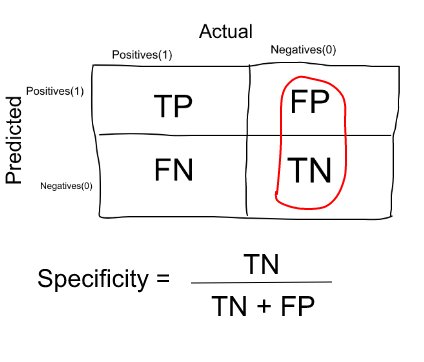Specificity is a measure that tells us what proportion of patients that did NOT have cancer, were predicted by the model as non-cancerous. The actual negatives (People actually NOT having cancer are FP and TN) and the people diagnosed by us not having cancer are TN. (Note: FP is included because the Person did NOT actually have cancer even though the model predicted otherwise).

Specificity is the exact opposite of Recall.

Ex: In our cancer example with 100 people, 5 people actually have cancer. Let’s say that the model predicts every case as cancer.

So our denominator(False positives and True Negatives) is 95 and the numerator, person not having cancer and the model predicting his case as no cancer is 0 (Since we predicted every case as cancer). So in this example, we can that that Specificity of such model is 0%.

### 6. F1 Score:

We don’t really want to carry both Precision and Recall in our pockets every time we make a model for solving a classification problem. So it’s best if we can get a single score that kind of represents both Precision(P) and Recall(R).

One way to do that is simply taking their arithmetic mean. i.e (P + R) / 2 where P is Precision and R is Recall. But that’s pretty bad in some situations.

Suppose we have 100 credit card transactions, of which 97 are legit and 3 are fraud and let’s say we came up a model that predicts everything as fraud. (Horrendous right!?)

Precision and Recall for the example is shown in the fig below.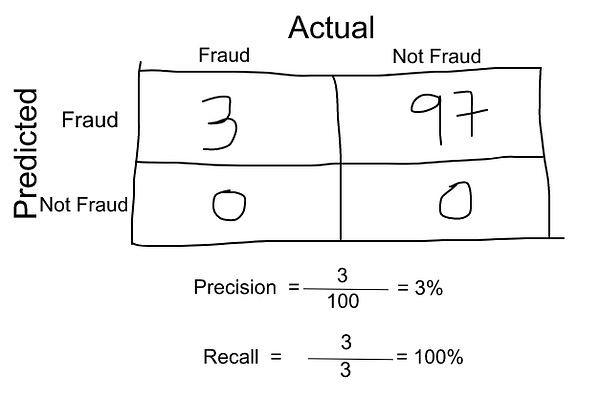Precision and Recall for Credit Card example

Now, if we simply take arithmetic mean of both, then it comes out to be nearly 51%. We shouldn’t be giving such a moderate score to a terrible model since it’s just predicting every transaction as fraud.

So, we need something more balanced than the arithmetic mean and that is harmonic mean.Arithmetic Mean vs Harmonic Mean

The Harmonic mean is given by the formula shown in the figure on the left.

Harmonic mean is kind of an average when x and y are equal. But when x and y are different, then it’s closer to the smaller number as compared to the larger number.

For our previous example, F1 Score = Harmonic Mean(Precision, Recall)

F1 Score = 2 * Precision * Recall / (Precision + Recall) = 2*3*100/103 = 5%

So if one number is really small between precision and recall, the F1 Score kind of raises a flag and is more closer to the smaller number than the bigger one, giving the model an appropriate score rather than just an arithmetic mean.

So far, we have seen what the Confusion matrix is, what is Accuracy, Precision, Recall (or Sensitivity), Specificity and F1-score for a classification problem. In the next post, I will be discussing the other metrics that can be used in Classification problems like the AUC-ROC Curve, Log loss, F-Beta score etc.

Till then stay tuned and Happy Machine Learning.